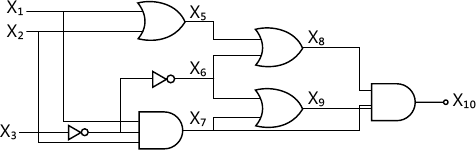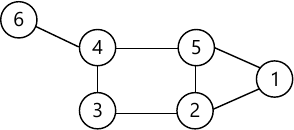MORE IN Design & Analysis of Algorithms
SPPU Computer Engineering (Semester 7)
Design & Analysis of Algorithms
December 2014
Total marks: --
Total time: --
INSTRUCTIONS
(1) Assume appropriate data and state your reasons
(2) Marks are given to the right of every question
(3) Draw neat diagrams wherever necessary

Answer any one question from Q1 and Q2
1 (a) Solve the recurrence relation
T (n) = T (n - 1) + T(n - 3) - T (n - 4), n> = 4 subject to T (n) = n for 0 < = n < = 3.
8 M
1 (b) Write an algorithm for insertion sort. State its time complexity.
6 M
1 (c) Explain with example the notations Big - O and Omega.
4 M

2 (a) Write Prim's algorithm for minimum spanning tree. Analyze the algorithm for time complexity.
8 M
2 (b) Explain Divide and conquer strategy with example of Binary Search
6 M
2 (c) Show that the following equality is correct 5n2 - 6n=θ (n2).
4 M

Answer any one question from Q3 and Q4
3 (a) Let n = 4 and (a1, a2, a3, a4) = (do, if, int, while), let p (1:4) = (3, 3, 1, 1) and q (0 : 4 ) = (2, 3, 1, 1), construct OBST using dynamic programming.
8 M
3 (b) What is dynamic programming? Define principle of optimality and explain it for 0/1 Knapsack.
8 M

4 (a) State multistage graphs problem and explain how it can be solved using backward approach.
8 M
4 (b) Find optimal solution for 0/1 Knapsack problem using Dynamic programming.
n=3, (W1, W2, W3)= (1,2,3) (P1, P2, P3)=(1,2,4) and m=6.
8 M

Answer any one question from Q5 and Q6
5 (a) Write an algorithm to solve 8-Queens problem using back tracking.
8 M
5 (b) Explain the steps of solving Travelling salesMan problem using Branch and Bound.
8 M

6 (a) Explain Graph colouring problem with respect to backtracking.
8 M
6 (b) What is Branch and Bound method? Explain FIFO Branch and Bound algorithm.
8 M

Answer any one question from Q7 and Q8
7 (a) Write Cook's algorithm in pseudo C and find out its time complexity. State the significance of this algorithm.
8 M
7 (b) Consider scheduling problem where six jobs have a profit of (10, 34, 67, 45, 23, 99) and corresponding deadlines (2, 3, 1, 4, 5, 3). Obtain optimum schedule. What is time complexity of your algorithm?
8 M

8 (a) Reduce the following circuit satisfiability to formula a satisfiability.6 M
8 (b) Define a Clique problem. Find out all possible Cliques for following graph. Does it contains a Clique of maximum size?6 M

Answer any one question from Q9 and Q10
9 (a) Explain pointer doubling algorithm with suitable example.
8 M
9 (b) How Quick sort can be implemented on multiprocessor system? Explain it with suitable Example.
8 M

10 (a) State and explain different parallel computational models.
8 M
10 (b) Write an algorithm for odd-even merge sort & Illustrate it with following set of numbers. 2, 4, 3, 5, 6, 1, 7, 8.
8 M

Answer any one question from Q11 and Q12
11 (a) Write an algorithm to implement Hoffman coding algorithm.
6 M
11 (b) What do you mean by Heuristic search algorithm? Explain it in brief with suitable example.
8 M
11 (c) State and explain Resource allocation algorithm
4 M

12 (a) State and explain Image edge detection algorithm.
8 M
12 (b) What is meaning of deadlock detection and deadlock avoidance ? What are the necessary conditions for deadlock to occur?
6 M
12 (c) Explain convex Hulls problem.
4 M

More question papers from Design & Analysis of Algorithms